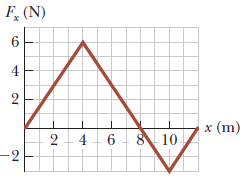# F (N)64x (m)8 102 46-2

Question

The force acting on a particle varies as shown in the figure below.

a) Find the work done by the force on the particle as it moves from x = 0 m to x = 8 m.

b) Find the work done by the force on the particle as it moves from x = 8 m to x = 12 m.

c) Find the work done by the force on the particle as it moves from x = 0 m to x = 12 m.help_outlineImage TranscriptioncloseF (N) 6 4 x (m) 8 10 2 4 6 -2 fullscreen
check_circleExpert Solution
Step 1

Work done by the force on the particle is equal to area under the force versus distance graph .

(a) Write the expression for work done by the force on the particle from x=0m to x=8m from the graph .

Step 2

(b) Write the expression for work done by the force on ...

### Want to see the full answer?

See Solution

#### Want to see this answer and more?

Solutions are written by subject experts who are available 24/7. Questions are typically answered within 1 hour*

See Solution
*Response times may vary by subject and question
Tagged in

### Work,Power and Energy